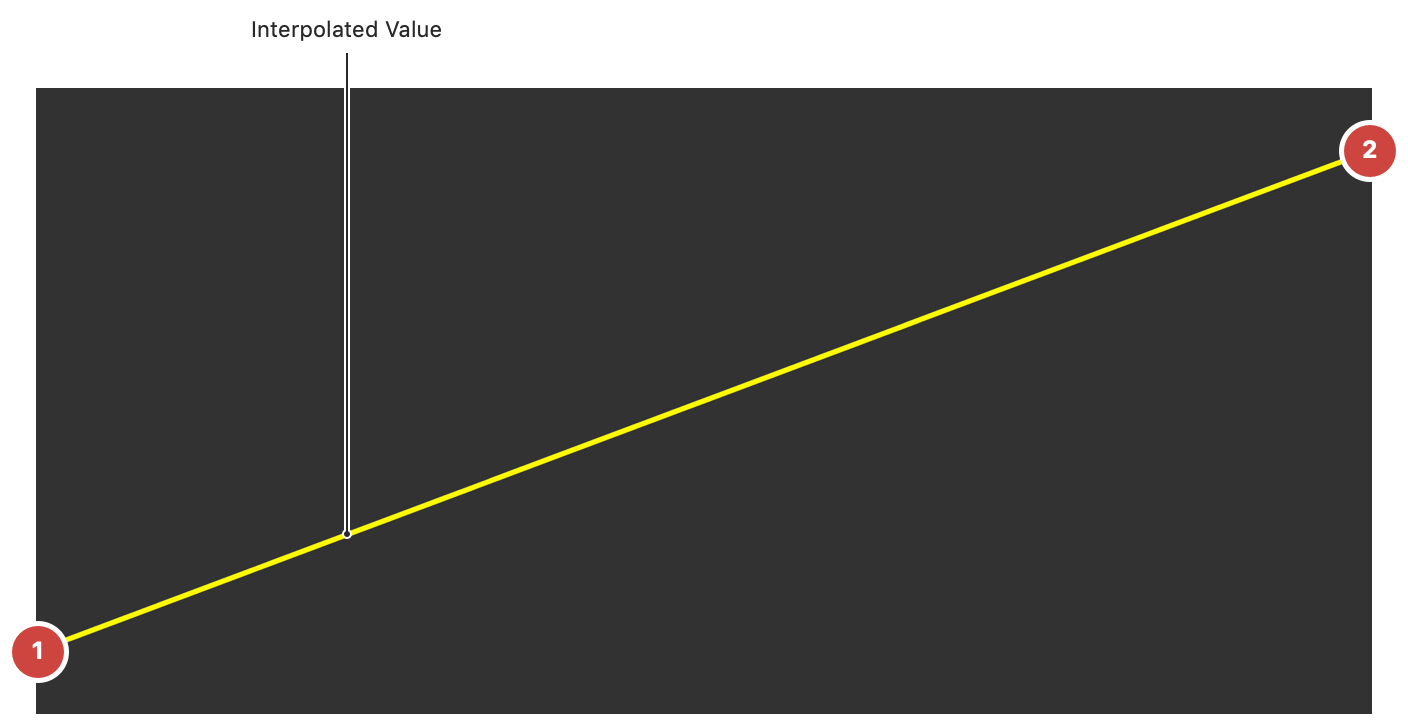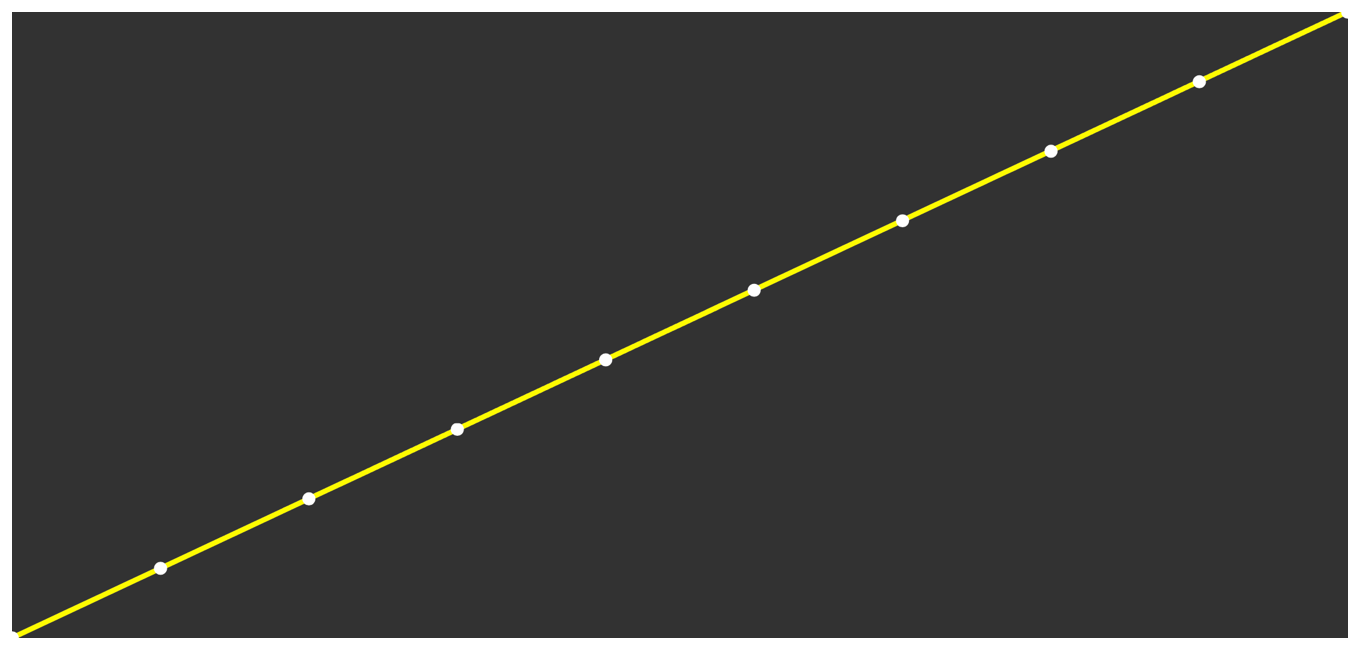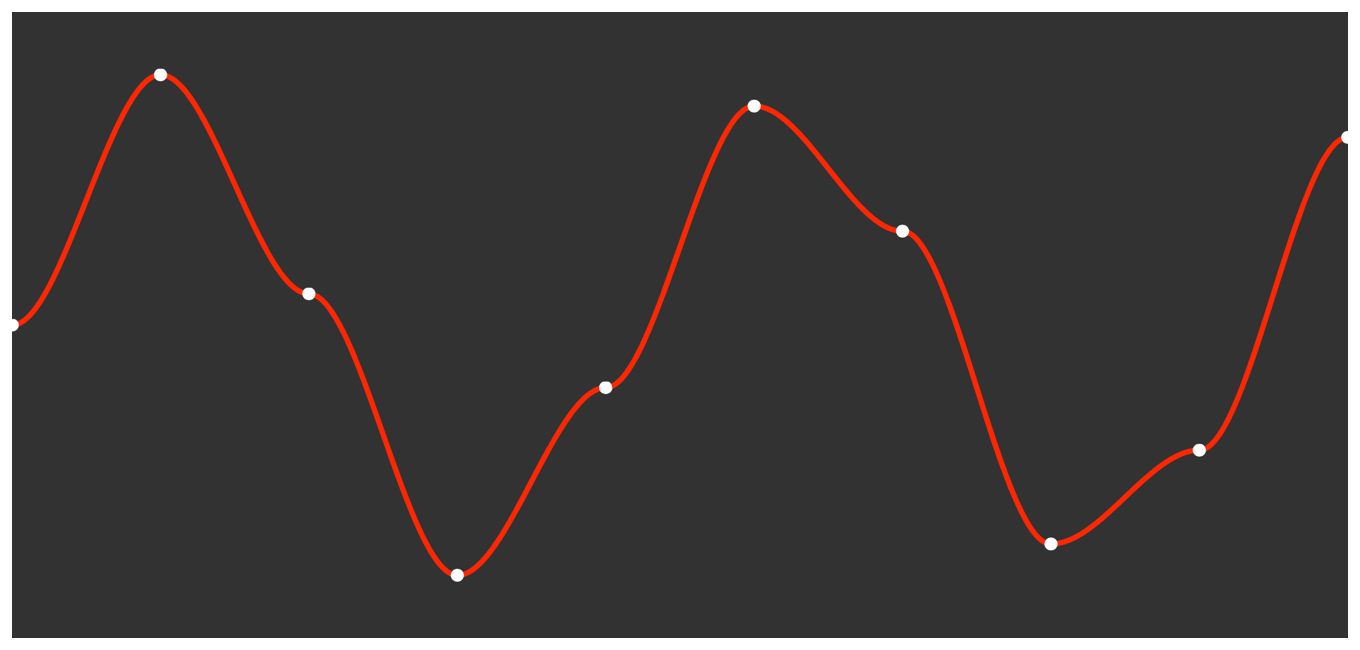Article

# Use Linear Interpolation to Construct New Data Points

Fill the gaps in arrays of numerical data using linear interpolation.

## Overview

Linear interpolation is a method of calculating intermediate data between known values by conceptually drawing a straight line between two adjacent known values. An interpolated value is any point along that line. You use linear interpolation to, for example, draw graphs or animate between keyframes. Figure 1 shows an example interpolated value between two points.Figure 1 A linearly interpolated value between values 1 and 2

vDSP provides two functions to linearly interpolate between the elements in an array.

This article discusses both functions to draw a continuous line graph based on a set of discrete data points.

### Create the Data

In this example, you'll interpolate the data represented by two arrays, `values` and `indices`:

Each array consists of 10 elements. A pair of corresponding elements in the two arrays defines a single point in the diagram as follows:

• Elements in the `values` array denote the vertical position.

• Elements in the `indices` array denote the horizontal position.Figure 2 A plot of the data points formed by the corresponding elements in values and indices

### Define Common Fundamental Constants

When defining the constants used to plot the points, this example uses `n` as the number of elements in the interpolation result, and `stride` as the stride for all arrays.

### Generate a Vector by Interpolation

The `vDSP_vgenp` function (and its double-precision equivalent, `vDSP_vgenpD`) accepts the values and indices arrays and an empty array to receive the interpolation result:

On return, `result` contains the interpolated values. Figure 3 shows the values in `result` as a line between the known values described by the `values` and `indices` arrays:Figure 3 A plot of data points generated by linear interpolation

### Interpolate With Fine Control

The `vDSP_vlint` and `vDSP_vlintD`) functions require an `indices` array that includes fractional parts. The fractional parts define the interpolation between the pair of values in the `values` array starting at the index defined by the integer part.

Use `vDSP_vgen` to generate a linear ramp from `0` to the number of elements in `values`, minus 1:

On return, `control` contains:

`         Float 0.0000`

`         Float 0.0087`

`         Float 0.0175`

`        ...`

`         Float 8.9824`

`         Float 8.9912`

`         Float 9.0000`

Figure 4 shows a visualization of the values in `control` with small circles indicating each integer index.Figure 4 A plot of the values in control generated with a linear ramp

With the control values defined, call `vDSP_vlint` to calculate the interpolated values:

On return, `result` contains the interpolated values. Figure 5 shows the values in `result` as a line between the known values described by the `values` and `indices` arrays.Figure 5 A plot of the linearly interpolated values based on a linear ramp

The result of `vDSP_vlint` is equal to the result of `vDSP_vgenp`.

### Add Smoothing to the Interpolation Result

You can change the way that you generate the control array to alter the interpolated result. For example, you may want to smooth the joins in a line graph or add easing to an animation. The following code uses `simd_smoothstep` to smooth the fractional parts of `control` near the increments to the integer parts:

Figure 6 shows a visualization of the values in `control` with small circles indicating each integer index.Figure 6 A plot of the values in control generated with smooth step

Using the same call to `vDSP_vlint` as above, the result, as shown in Figure 7, shows a smoother transition between the known values.Figure 7 A plot of the linearly interpolated values based on smooth step

### Signal Processing Essentials

Controlling vDSP Operations with Stride

Operate selectively on the elements of a vector at regular intervals.

Using vDSP for Vector-based Arithmetic

Increase the performance of common mathematical tasks with vDSP vector-vector and vector-scalar operations.

Resampling a Signal with Decimation

Reduce the sample rate of a signal, by specifying a decimation factor and applying a custom antialiasing filter.

vDSP

Perform basic arithmetic operations and common digital signal processing routines on large vectors.